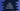# Java Program to Multiply Two Matrices## Java Program to Multiply two matrices :

In this Java example, we will learn how to write a program to multiply two matrices. First we will take inputs of both the matrices from the user. Then we will multiply both matrices and print out the result. For taking inputs from the user , multiply matrices and to print a matrix, we have different methods.

We can multiply two matrices if and only if the number of columns of the first matrix equals the number of rows in the second matrix. So,the result of one 2? matrix with a 4? matrix will be a matrix of size 2?

### Steps we are using in the program :

1. First, take the row and column counts for the first matrix from the user.

2. Take the inputs for the first matrix from the user using ‘getInputsForMatrix’ method.

3. Similarly, take the row, column counts and inputs of the second matrix similarly.

4. Multiply both matrices using ‘multiply’ method. It returns a result matrix containing the multiplication of both the matrices.

5. Print out the result matrix using ‘printMatrix’ method.

### Java Program :

``````import java.util.Scanner;

public class Main {

/**
* Utility functions for System.out.println() and System.out.print()
*/
private static void print(String str) {
System.out.print(str);
}

private static void println(String str) {
System.out.println(str);
}

/**
* Function to print a matrix
*
* @param matrix : Matrix to print
* @param row    : row count of the matrix
* @param column : column count of the matrix
*/
private static void printMatrix(int[][] matrix, int row, int column) {
for (int i = 0; i < row; i++) {
for (int j = 0; j < column; j++) {
print(matrix[i][j] + " ");
}
println("");
}
}

/**
* Get inputs for a matrix
*
* @param matrix : inputs will be save in this matrix
* @param row    : row count for the matrix
* @param column : column count of the matrix
*/
private static void getInputsForMatrix(int[][] matrix, int row, int column) {
Scanner scanner = new Scanner(System.in);

for (int i = 0; i < row; i++) {
for (int j = 0; j < column; j++) {
matrix[i][j] = scanner.nextInt();
}
}

}

//multiply two matrix
private static int[][] multiply(int[][] matrix1, int[][] matrix2, int row1, int column1, int row2, int column2) {
int[][] resultMatrix = new int[row1][column2];

for(int i = 0; i < row1; i++) {
for (int j = 0; j < column2; j++) {
for (int k = 0; k < column1; k++) {
resultMatrix[i][j] += matrix1[i][k] * matrix2[k][j];
}
}
}

return resultMatrix;
}

public static void main(String args[]) {
int row1;
int column1;
int row2;
int column2;

Scanner scanner = new Scanner(System.in);

//get inputs for first matrix
println("Enter row of first matrix : ");
row1 = scanner.nextInt();
println("Enter column of first matrix : ");
column1 = scanner.nextInt();

int[][] matrix1 = new int[row1][column1];
println("Enter the First Matrix :");
getInputsForMatrix(matrix1, row1, column1);

//get inputs for second matrix
println("Enter row of second matrix : ");
row2 = scanner.nextInt();
println("Enter column of second matrix : ");
column2 = scanner.nextInt();

int[][] matrix2 = new int[row2][column2];
println("Enter the second Matrix :");
getInputsForMatrix(matrix2, row2, column2);

//calculate the multiplication
int[][] resultMatrix = multiply(matrix1, matrix2, row1, column1, row2, column2);

println("First matrix * Second Matrix : ");

//print result matrix
printMatrix(resultMatrix, row1, column2);

}
}
``````

### Sample Output :

``````Enter row of first matrix :
3
Enter column of first matrix :
2
Enter the First Matrix :
1 2 3
4 5 6
Enter row of second matrix :
2
Enter column of second matrix :
2
Enter the second Matrix :
1 2
1 3
First matrix * Second Matrix :
3 8
7 18
11 28
``````